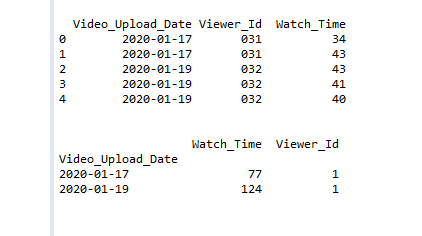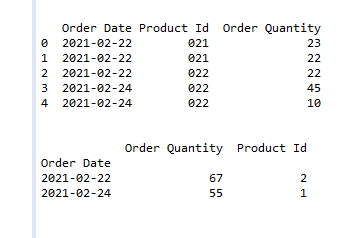Related Articles

# Count distinct in Pandas aggregation

• Last Updated : 03 Mar, 2021

In this article, let’s see how we can count distinct in pandas aggregation. So to count the distinct in pandas aggregation we are going to use groupby() and add() method.

• groupby(): This method is used to split the data into groups based on some criteria. Pandas objects can be split on any of their axes. We can create a grouping of categories and apply a function to the categories. The abstract definition of grouping is to provide a mapping of labels to group names
• agg(): This method is used to pass a function or list of functions to be applied on a series or even each element of series separately. In the case of a list of functions, multiple results are returned by agg() method.

Below are some examples which depict how to count distinct in Pandas aggregation:

Attention geek! Strengthen your foundations with the Python Programming Foundation Course and learn the basics.

To begin with, your interview preparations Enhance your Data Structures concepts with the Python DS Course. And to begin with your Machine Learning Journey, join the Machine Learning - Basic Level Course

Example 1:

## Python

 `# import module``import` `pandas as pd``import` `numpy as np`` ` `# create Data frame``df ``=` `pd.DataFrame({``'Video_Upload_Date'``: [``'2020-01-17'``,``                                         ``'2020-01-17'``,``                                         ``'2020-01-19'``,``                                         ``'2020-01-19'``,``                                         ``'2020-01-19'``],``                   ``'Viewer_Id'``: [``'031'``, ``'031'``, ``'032'``,``                                 ``'032'``, ``'032'``],``                   ``'Watch_Time'``: [``34``, ``43``, ``43``, ``41``, ``40``]})`` ` `# print original Dataframe``print``(df)`` ` `# let's Count distinct in Pandas aggregation``df ``=` `df.groupby(``"Video_Upload_Date"``).agg(``    ``{``"Watch_Time"``: np.``sum``, ``"Viewer_Id"``: pd.Series.nunique})`` ` `# print final output``print``(df)`

Output:Example 2:

## Python

 `# import module``import` `pandas as pd``import` `numpy as np`` ` `# create Data frame``df ``=` `pd.DataFrame({``'Order Date'``: [``'2021-02-22'``,``                                  ``'2021-02-22'``,``                                  ``'2021-02-22'``,``                                  ``'2021-02-24'``,``                                  ``'2021-02-24'``],``                   ``'Product Id'``: [``'021'``, ``'021'``,``                                  ``'022'``, ``'022'``, ``'022'``],``                   ``'Order Quantity'``: [``23``, ``22``, ``22``,``                                      ``45``, ``10``]})`` ` `# print original Dataframe``print``(df)`` ` `# let's Count distinct in Pandas aggregation``df ``=` `df.groupby(``"Order Date"``).agg({``"Order Quantity"``: np.``sum``,``                                   ``"Product Id"``: pd.Series.nunique})`` ` `# print final output``print``(df)`

Output:My Personal Notes arrow_drop_up